# Team:Aberdeen Scotland/Bifurcation

(Difference between revisions)
 Revision as of 14:22, 23 October 2010 (view source)Brychan (Talk | contribs)← Older edit Revision as of 14:22, 23 October 2010 (view source)Brychan (Talk | contribs) Newer edit → Line 23: Line 23:
-

2. find the fixed points of the system of differential equations:

+

2. Find the fixed points of the system of differential equations:

There is a fixed point at x=y=0.  By observation, we can see that there are fixed points at x=0, y=2, and x=3, y=0.  Finally, by solving this system of equation, we see that there is also a fixed point at x=1 and y=1.

There is a fixed point at x=y=0.  By observation, we can see that there are fixed points at x=0, y=2, and x=3, y=0.  Finally, by solving this system of equation, we see that there is also a fixed point at x=1 and y=1.

## Revision as of 14:22, 23 October 2010

University of Aberdeen - ayeSwitch - iGEM 2010

iGEM 2010

# Bifurcation Analysis

The literal meaning of bifurcation is, "division into two parts, branches or aspects" (Answers). In mathematics, this 'division' is reference to when non-linear equation suddenly switches from having one solution to having multiple solution when a parameter of the system is varied. The point at which bifurcation occurs is referred to as the 'bifurcation point.' If a system is bistable it can be stable, or at rest in two distinct states.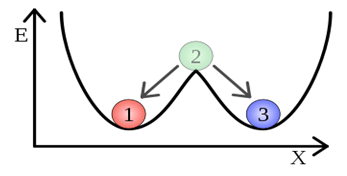Figure 1.This is a graphical representation of bistability. Ball 2 ban be equally 'happy' in either the 'one' or 'three' positions. Once it falls into one of these states, ball 2 will not be inclined to leave this state, hence making it a "stable state."

### Why are they important?

Analysing the stability of a system is a key to the success of a project. In biological terms, bistability represents the potential for out system to switch between the expression of green and cyan fluorescent proteins. Our goal is to analyse the parameter space in order to determine which parameters are needed to allow for bistability in the system.

a bifurcation diagram has branches which define the possible states a system can rest in. By analysing the bifurcation plots, we can determine how far apart the two "branches," or stable states, are. The further apart the branches, the harder it will be to attain a switch between the two stable states, however the switch also becomes more stable with increased distance between the branches. Therefore, we want to manipulate the parameters in the system to guarantee stable bistability.

### How do you analyse the stability of a system?

In order to analyse the stability of the system, we utilised XPP Auto, C programming, and MATLAB. we will attempt to detail the math behind what the programme does computationally. The process involved with general stability analysis is as follows. We have takes a simple example system that is easy to analyse in order to demonstrate the general process:

Take the following example system of two differential equations: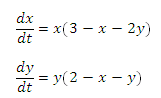#### 1. Set the differential equations to zero: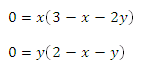#### 2. Find the fixed points of the system of differential equations:

There is a fixed point at x=y=0. By observation, we can see that there are fixed points at x=0, y=2, and x=3, y=0. Finally, by solving this system of equation, we see that there is also a fixed point at x=1 and y=1.

#### 3. Evaluate the Jacobian matrix of the system at these fixed points:

If we label the top function f1 and the bottom f2, the Jacobian matrix of the system will be of the form: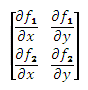When we evaluate the Jacobian matrix for our example system of equations, we get: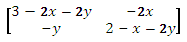#### 4. Using the Jacobian matrix, find the corresponding eigenvalues:

Definition: An eigenvalue is a value λ such that Aλ=λx, where x is said to be an eigenvector of A. In order to solve for λ, solve the following equation: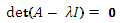where det is the determinant of the matrix and I is the identity matrix. This is known as the the characteristic equation of a matrix.

In the case of our example system, the eigenvalues are: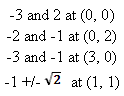#### 5. Take the signs of these eigenvalues in order to analyse stability:

The eigenvalues of a system give important information about the stability of the system. The stability of each of the fixed points can be analysed based on the following criteria:

 Positive real and complex parts : Unstable Negative real and complex parts : Stable Positive real part : Unstable spiral (spiraling out) Negative real part : Stable spiral (spiraling inwards)

So, for our example system, it can be deduced that the stability at the fixed points is as follows:

One positive and one negative eigenvalue at (0,0) means that (0,0) is a saddle node
Two negative eigenvalues at (0,2) means that (0,2) is a stable node
Two negative eigenvalues at (3,0) means that (3,0) is a stable node
One positive and one negative eigevalue at (1,1) means that (1,1) is a saddle node